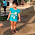## Saturday, 23 August 2014

### Example for Apriori Algorithm

Lets take a store data
```pen,pencil
pencil,book,eraser
pen,book,eraser,chalk
pen,eraser,chalk
pen,pencil,book
pen,pencil,book,eraser
pen,Ink
pen,pencil,book
pen,pencil,eraser
pencil,book,chalk```
Step 1: Initially we need to find Item 1 Frequent Dataset
```c1
------
book 6
chalk 3
eraser 6
pen 8
pencil 7
Ink 1```
We will say that an item set is frequent if it appears in at least 3 transactions of the itemset: the value 3 is the support threshold.

Support count = 3 (user defined)

So the items less that support count can be discarded form F1 frequent Dataset.
so our new set will be
```L1
------
book 6
chalk 3
eraser 6
pen 8
pencil 7```
Step 2: We need to generate size 2 frequent item pair sets by joining L1 set
eg:{book} U {chalk} => {book,chalk} and so on..
```{book,chalk}
{book,eraser}
{book,pen}
{book,pencil}

{chalk,eraser}
{chalk,pen}
{chalk,pencil}

{eraser,pen}
{eraser,pencil}

{pen,pencil}```
Once the transactions are joined we need to identify the no occurence of the above data items in original transaction(That will be the support count of C2)
```C2
----------------
{book,chalk} 2
{book,eraser} 2
{book,pen} 4
{book,pencil} 5

{chalk,eraser} 2
{chalk,pen} 2
{chalk,pencil} 0

{eraser,pen} 5
{eraser,pencil} 3

{pen,pencil} 5
```
Transactions less that support count can be discarded form C2 frequent Dataset
```L2
----------------
{book,pen} 4
{book,pencil} 5
{eraser,pen} 5
{eraser,pencil} 3
{pen,pencil} 5```
To find C3 loop through L2
eg: {book,pen} U {book,pencil} => {book,pen,pencil}
```C3
-------------------------
{book,pen,pencil} 3
{chalk,eraser,pen} 2
{eraser,pen,pencil} 2```
Transactions less that support count can be discarded form C3 frequent Dataset
```L3
-------------------------
{book,pen,pencil} 3```
There are no transaction to join further.
So our Frequent item sets are
```L1:
-------
book 6
chalk 3
eraser 6
pen 8
pencil 7

L2:
-----------------
{book,pen} 4
{book,pencil} 5
{eraser,pen} 5
{eraser,pencil} 3
{pen,pencil} 5

L3
-------------------------
{book,pen,pencil} 3```
Step 3: We need to generate Strong Assosiaction  Rules for frequent Set using L1,L2and L3

Say confidence is 60% and Support count is 3.So we have to find the Transactions with no.of item 3  and which has a confidence >=60.Now we can identify L3 set
`{book,pen,pencil} 3`

Finding Ruleset
```{book,pen} => pencil
{book,pencil} => pen
{pen,pencil} => book

pencil => {book,pen}
pen => {book,pencil}
book => {pen,pencil}
```
Now we need to find the confidence of each transaction
```eg: {book,pen} => pencil
= support Cnt{book,pen,pencil}/ support count({pencil})
```

Therefore rules having confidence greater than and equal to 60 are
```book,pen=>pencil 75.0
book,pencil=>pen 60.0
pen,pencil=>book 60.0```
These are the strongest rules.
If a customer buys book and pen he have a tendency to buy a pencil too. Like wise if he buys book and pencil he may buy pen too.

1.please provide code in java

thanks
siva'
9030865822

2.{book,pen} => pencil
= support Cnt{book,pen}/ support count({pencil})
= 4 / 8 = 50% which is lesser than 60%

3.Very much useful article. Kindly keep blogging

Java Training in Chennai

Java Online Training India